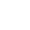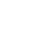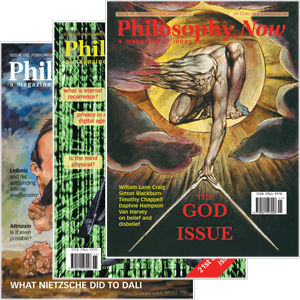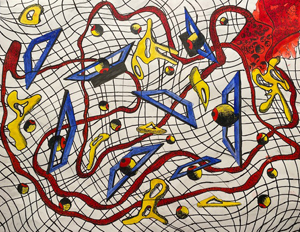# Is Mathematics in the Fabric of the Universe?

Is mathematics invented or discovered? Do numbers exist? Many mathematicians tend towards versions of mathematical Platonism, broadly construed. This means that in one way or another, occasionally explicitly, but mostly implicitly, they presuppose that mathematics is in some sense inherent in reality, existing independently of the human mind. Eminent figures holding the view include Kurt Gödel, Roger Penrose, Alain Connes, Albert Lautman, and Hugh Woodin, to name but a few. This article aims to show them to be (mostly) wrong about this. I will discuss the idea by concentrating on the simplistic popular version of mathematical Platonism beloved of school teachers – the idea that ‘mathematics is contained within the fabric of the universe’.

One popular way in which this idea is supported is through the argument that plants and animals are physical instantiations of the Fibonacci Sequence. Cruder versions of the argument often appeal to the Golden Ratio appearing in nature.

The Fibonacci Sequence is a series of numbers built through the rule that in order to find the next term in the sequence you must add the previous two terms. This yields 1, 1, 2, 3, 5, 8, 13, 21, 34… and so on. It is a sequence said to appear widely in nature – in ammonites, flowers, pine cones, and more – and in this sense is supposed to show that mathematics is contained within the fabric of the universe.

Using the Golden Ratio to support this same conclusion is perhaps less compelling. The relative proportions of physical objects can be relatively pleasing or displeasing to the eye, and one obvious question to ask is why this might be. Mathematicians, along with philosophers and scientists, have made varying attempts to answer it. Euclid is generally accepted to have provided an answer by saying that the Golden Ratio is the perfect harmonious ratio. It’s the ratio where, in a line made of length A plus length B, the ratio of these lengths is (A+B)/A=A/B. It comes out to about 1.618. Since the heyday of Classical Greece this ratio has been routinely expressed in architecture and art, and its importance in mathematics has led to it being given its own Greek letter, ϕ (phi). There are even apps which use it to let you know how attractive you are!

The relationship between these aspects of mathematics and the empirical world is well known, and often believed to be a justification for the thought that mathematics is inherent in the fabric of the universe. I hope to show this justification to be hollow.Pattern image © Paul Gregory 2021

## Stable & Unstable Meanings

I will concentrate on two dimensions of the logic of mathematics. The first dimension is that convention determines which concepts are stable – for example, numbers – and which are not – for example, political concepts such as ‘right wing’, ‘left wing’ and ‘undemocratic’. Stipulations of the meaning of concepts result from linguistic rule-following and practice, and even the most stable of the meanings of concepts is still a matter of convention. The second dimension is the distinction between logical determination and causal determination – that is, the distinction between a concept being necessary or it being contingent. So ‘a triangle has three sides’ is necessarily true because of the meanings of the terms of the proposition, and highly stable precisely because there is no dispute concerning the meanings of those terms. The same is true of other necessary propositions such as 2+3=5, or that 34 succeeds 21 in the Fibonacci Sequence.

In an important sense, however, the necessary natures of these propositions is dependent upon context. For example, were I teaching a child the meaning of the term ‘triangle’, then the predicate ‘has three sides’ would not be internal to or contained within the subject, that is, necessarily implied by the word ‘triangle’. Rather, in this kind of context, the predicate tells the child something new about the subject. Similarly, the number and other terms need to be understood before 2+3=5 can be understood as a necessary proposition. Once understanding of the meaning of the terms is achieved, however, no recourse to experience is required to verify such pure mathematical propositions.

In this definitional sense, even necessary mathematical propositions are conventions of geometry and mathematics. However, there is stability of meaning in relation to the terms used. When I talk about a triangle or use number terms, I do not confer meaning on them each time I use them. Rather, I show their conventional meaning in my use of mathematics and geometry. Put another way: the giving of a definition for any term, or the stipulation of a rule (such as for the Fibonacci Sequence), is itself neither true nor false, but purely contingent: the same signs didn’t have to mean what they conventionally actually do mean. However, once the meaning of the terms has been established, the tautologous, necessary truth of the proposition ‘a triangle has three sides’, or of ‘2+3=5’ becomes evident.

That I do not give the meanings to the terms myself shows an interconnectedness between public practice and truth and falsity. The ‘public practice’ aspect is that when I say ‘‘A triangle has three sides’’ I am speaking English, and using terms that have clear and accepted meanings and uses in geometry and mathematics. The proposition is, therefore, rendered true by English language practices in a way that ensures one does not need further recourse to experience in order to establish its truth-value. Rather, the proposition is true simply because the terms have been publically defined to mean what they do. This means that there is a logical connection between our concepts and our practices, including the stipulations we provide to govern the use of these concepts - these are also practices. So one is only doing mathematics if one fulfils certain criteria in practice – for instance, accepting the meanings of the terms involved in ‘2+3=5’. The proposition would be false or nonsense if one or more of its terms meant something other than it does. It is the stability of the established meanings of the terms which ensures this is not the case – and such stability is dependent upon our linguistic practices.

By contrast, the arguments which surround what is deemed ‘undemocratic politics’ are wrapped up with a lack of agreement over the meaning of the term. In other words, it is because the meaning of ‘undemocratic’ is not universally agreed upon that many arguments occur. Stipulations of meaning (the creation of definitions) cannot be true or false, but nevertheless need to be generally accepted and stable if the propositions in which they are contained are to be truth-valued. But there are many varieties of political set-up in which there is an electorate which are on occasion termed ‘undemocratic’, some of which are incompatible with one another; for example, ‘first past the post’ versus ‘proportional representation’. For this reason there exists no way in which propositions that contain the term ‘undemocratic’ can be absolutely truth-valued. After all, there exists ‘The Democratic People's Republic of Korea’ – which shows both that the term ‘democratic’ does not have a clearly-accepted universal meaning, and that what counts as democratic or undemocratic practice is often not settled.

The relationship between linguistic meaning and human practices is also clear here and obviously interdependent. But unlike the term ‘triangle’ or the number 2, the term ‘undemocratic’ cannot be used with anything like mathematical accuracy. Instead, within political arguments there are differing definitions and uses of the same term taking place simultaneously, amongst a variety of political set-ups. What is ‘democratic’ or ‘undemocratic’ cannot, therefore, refer to one particular kind.

## The Language of MathematicsFibonacci Flower © Anna Benczur 2015

For those who maintain that mathematics is in the fabric of the universe, the stability that exists in mathematical propositions is understood to exist in the nature of things - in features such as ideal shapes or ratios: perfect circles and so on. Empirical justification has been attempted for this kind of mathematical Platonism: we can see these perfect forms expressed in imperfect copies in nature – in circles, plant cells, etc. As mentioned, it is also thought that there’s a necessary relationship between certain shapes in nature and such pure mathematical concepts as the Fibonacci Sequence or the Golden Ratio.

Genuine mathematical knowledge is held to be absolute and beyond any empirical justification, which is vulnerable to error. Thus, it is believed, the stringency of justification needed to attain mathematical knowledge needs to be higher than that for knowledge gained through observation. Nevertheless, mathematical perfection is visible within nature, the argument goes. So whilst mathematical knowledge requires a chain of error-free justification, it is subsequently quite possible to observe that mathematical perfection in nature, and to understand it as such. So – this thought continues – it is demonstrable that mathematics is inherent in the fabric of the universe.

However, an important observation needs to be made here in relation to mathematical propositions (for example, 2+3=5) and empirical propositions which can be expressed mathematically (for example, Newton’s second law of motion, which can be expressed as F=ma). The observation is that a mathematical proposition such as 2+3=7 is not a mathematical proposition. Granted, it bears a resemblance to such a proposition because of its use of numbers and other mathematical terms, but it is necessarily false. Accordingly, there is never a possibility that it can be true.

This is not the case with empirical propositions. It is logically possible for the structure of the universe to have been (or be) quite different from the way it is with different physical laws and so on. In this sense, it is, at least in principle, possible for Newton's second law of motion to be false if the structure of the universe were other than the way it is. So it seems that an empirical proposition can be false but remain an empirical proposition, whereas a mathematical proposition cannot be false and remain a mathematical proposition.

But why can 2+3=5 not be otherwise? What makes it immutably, necessarily true?

In order to correctly say that we are doing mathematics we need to follow mathematical rules. Much as we are required to follow certain rules in order for it to be correctly said that we are playing chess, so the same is true of mathematics. If we say ‘2+3=7’, then, clearly, the mathematical rules are not being followed (this is a further reason why it is not a mathematical proposition).

We can therefore say two things. Firstly, the rules required in order to say that we’re doing mathematics or playing chess express the essence or the fundamental dimensions of that activity. Secondly, following and developing rules (of any kind) is a human activity. These rules do not exist independently of humanity.Consider both of these claims in relation to rules that change – such as the rules of cricket, which have seen regular changes since the first test match was played back in 1877. We now have ‘50 over cricket’, ‘20-20 cricket’, and the brand new ‘the hundred’. Had these new formats been shown to the cricket-playing nations of the late nineteenth and early twentieth centuries, they would doubtless have said that they were ‘not cricket’. The developments and changes that have characterized the game are human activities, and, whatever one’s opinion of those changes, there needs to be agreement in practice between the players. This requires an understanding of the rules. So the rules, firstly, express the essence of the game of cricket, and, secondly, show it to be an entirely human practice, entirely the result of human stipulations.

When we share rules, we share practices. If we did not agree upon the rules of chess, then we would not be able to play chess. Yet, as Ludwig Wittgenstein put it, this is not a question of an agreement of opinion – it is not merely my opinion that these are the rules of chess or cricket. Rather, playing cricket or chess is a shared practice and the shared practice itself provides the criteria for saying whether or not the rules have been followed correctly. One can only be right or wrong about the rules of cricket, chess, or mathematics because they’ve already been defined. So the practices themselves are not opinions, even though opinions can be expressed in relation to them (for example, I might object to 20-20 cricket).

What we have when we follow rules is not agreement in opinion, then, but rather, agreement in judgement, or, to use Wittgenstein’s language again, agreement in form of life or practice. The uniformity of the rules of mathematics – the fact that they express a form of human practice that is universally shared – provides a stable framework through which we are able to describe reality; the Fibonacci Sequence and Golden Ratio being two examples where we do so. The necessity of mathematical propositions is based on the agreed rules as to what mathematical terms mean in much the same way that rules in chess require public agreement in order for the game of chess to exist and be played at all. In this way the essence of mathematics is determined by agreement in judgement concerning mathematical terms. Put another way: the conceptual structure which we use to describe the world is dependent on the criteria we use for saying we have applied our concepts correctly or incorrectly. The rules that govern the application of mathematical concepts may be used to express the essence of the world; but that does not mean that such rules are contained within the fabric of the universe itself.

Rules require certain things of us, but that is no reason to infer that the relationship between rule and practice is causal. If such a relationship were causal it would make our mathematical practices contingent, as it is always possible for a causal relationship not to hold in any given instance (the relationship is not necessary). Were the relationship between mathematical rules and practices contingent, it would be possible for the relationship between a mathematical rule and its application not to hold in any given instance, as this would be an empirical rather than a logical matter. For example, a contingent mathematics would mean that it was possible that 2+3 not equal 5 in a given instance; that sometimes 2+3 might equal 7. However, the fact that one only follows a rule in mathematics if one fulfils certain practices instead shows there to be a logical connection between our mathematical rules, practices, and concepts. If our practices did not exist – if human beings did not exist – then mathematical practices would not exist, and, accordingly, neither would the mathematical rules which are the criteria for saying whether or not mathematics is being done. The rules of mathematics are not, therefore, in the fabric of the universe, existing independently of human beings. So, since the rules of an activity are the essence of that activity, mathematics cannot be held to be in the fabric of the universe.

But here’s a final thought: human beings do exist: we are creatures in the universe, and we have developed forms of life. These forms of life are interdependent with our practices, which practices, in turn, provide the criteria for determining both the necessity of mathematical propositions and whether we have applied mathematical rules correctly. Certainly, it is a contingency that human beings exist – much as it is a contingency that the rest of the universe exists; but that we do exist with our mathematical practices perhaps shows that mathematics is inherent in the fabric of the universe after all!